5100

Physics Wave Optics Level: Misc Level

A ray of light in material # 1 strikes an interface at an angle of 40 dregrees, and the ray of light enters material # 2. In the NEW material, is the speed of light (A) LESS THAN (B) GREATER THAN (C) THE SAME AS, the speed of material # 1?. Or, does the answer depend on the frequency of the light, or is more information needed to be able to answer this question? Explain your answer.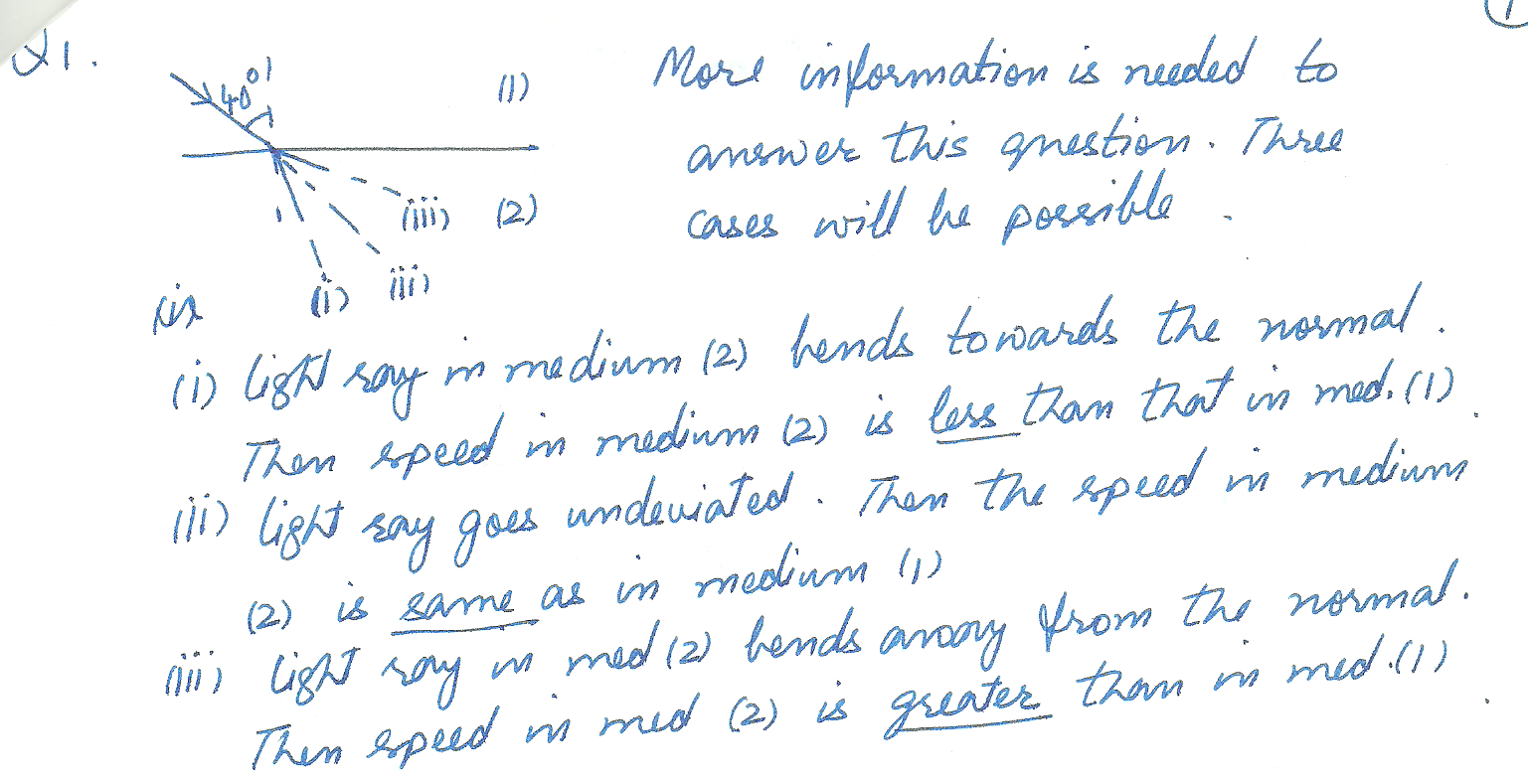5075

Physics Wave Optics Level: Misc Level

Electrons with K =100 eV are directed at two narrow slits a distance

d apart. If the angle between the central maximum of the resulting interference

pattern and the next maximum is to be one degree, what should d be?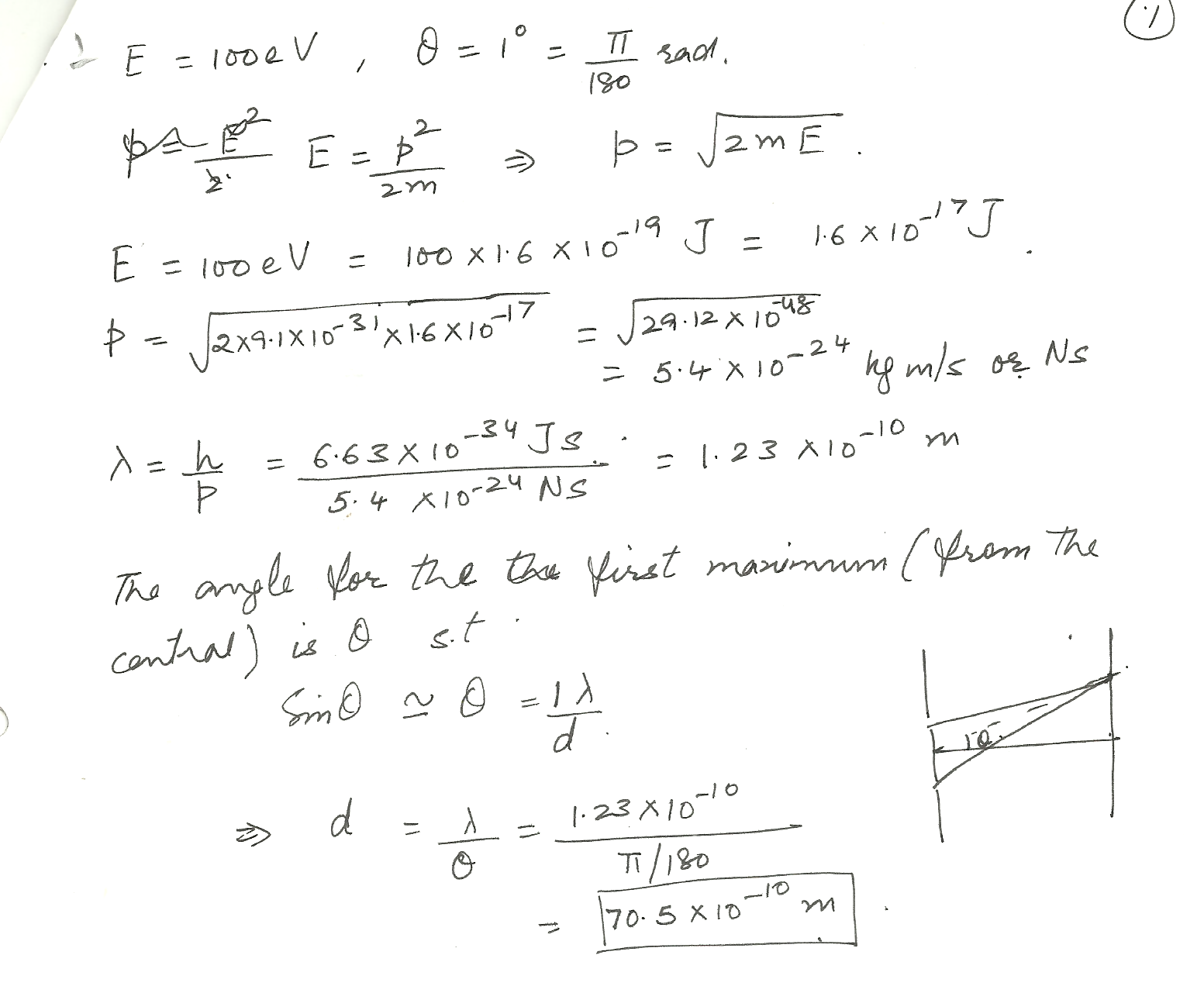5074

Physics Wave Optics Level: Misc Level

For diffraction by a single slit, what is the effect of increasing (a) the slit width, and (b) the wavelength?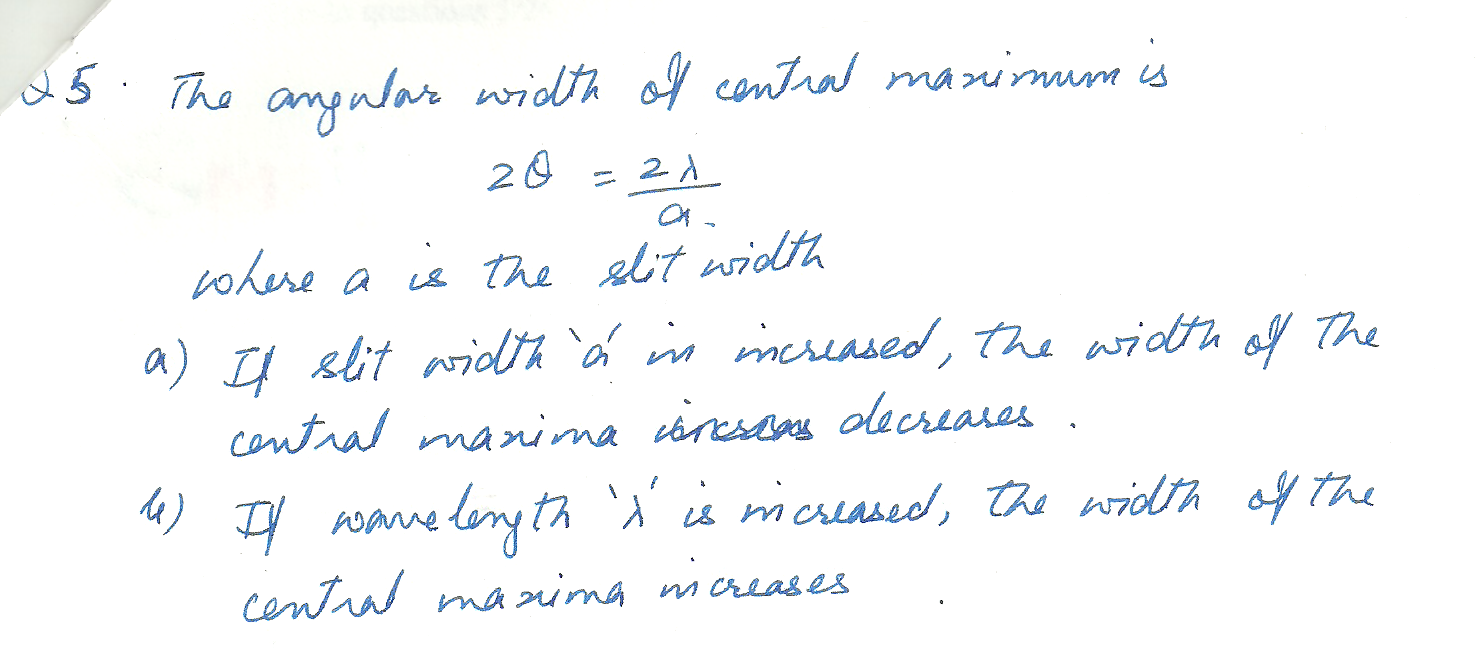5072

Physics Wave Optics Level: Misc Level

Compare a double- slit experiment for sound waves to that for light waves. Discuss the similarities and differences4965

Physics Wave Optics Level: Misc Level

Calculate the wavelength in nanometers of green light that has a frequency of 5 x10 Hz.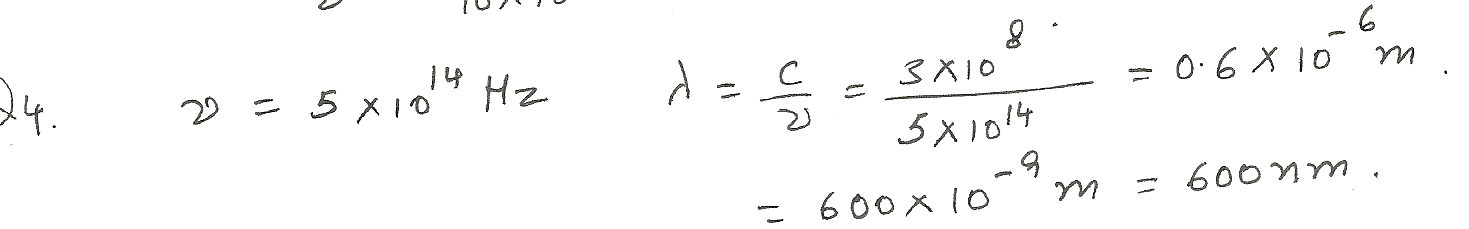4770

Physics Wave Optics Level: Misc Level

In a Young s double - slit experiment, a set of parallel slits with a separation of 0.100 mm is illuminated by light having a wavelength of 589 nm and the interference pattern observed on a screen 4.00 m from the slits. (a) What is the difference in path lengths from each of the slits to the screen location of a third -order bright fringe? (b) What is the difference in path lengths from the two slits to the screen location of the third dark fringe away from the center of the pattern?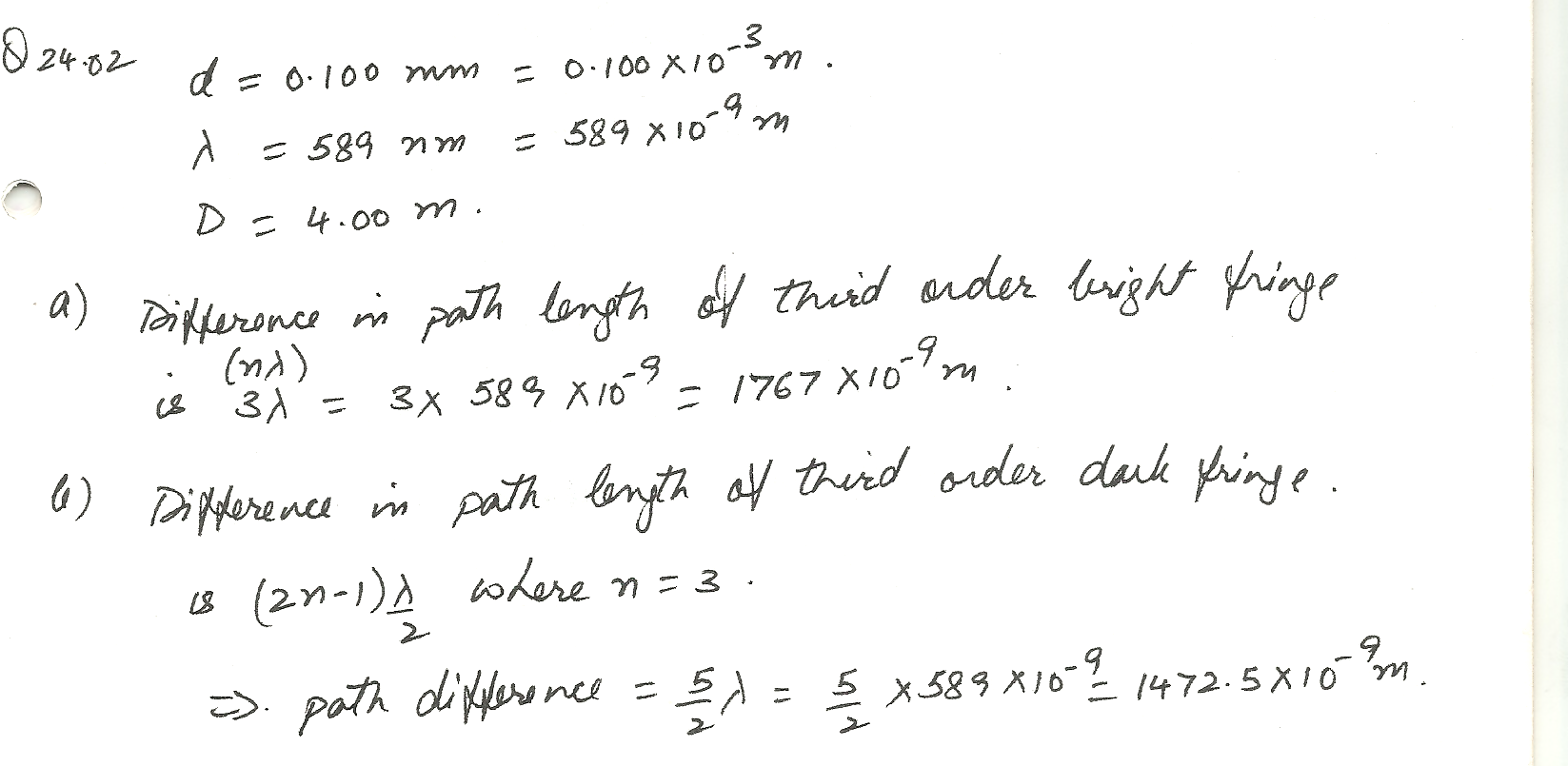4769

Physics Wave Optics Level: Misc Level

White light is spread out into its spectral components by a diffraction grating. If the grating has 2 000 lines per centimeter, at what angle does red light of wavelength 640 nm appear in the first -order spectrum?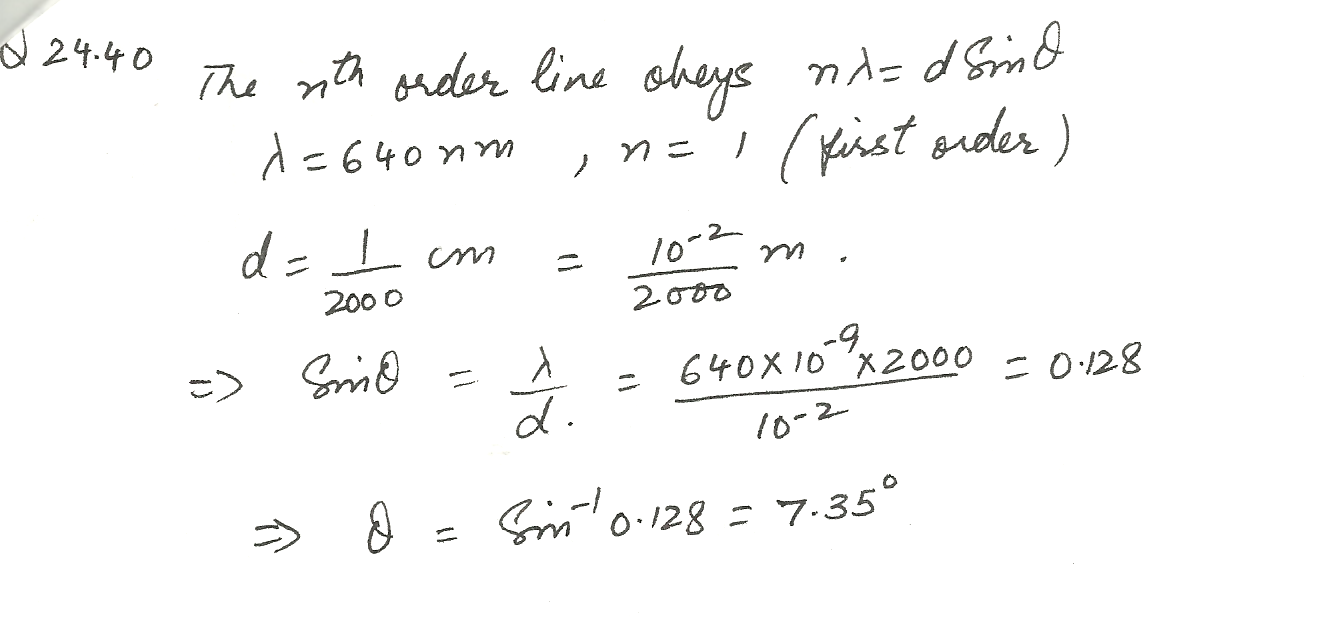3009

Physics Wave Optics Level: High School

Dual Nature of Light

An owl has good night vision because its eyes can detect a light intensity as small as 5.0 10-13 W/m2 . What is the minimum number of photons per second that an owl eye can detect if its pupil has a diameter of 7.0 mm and the light has a wavelength of 545 nm ?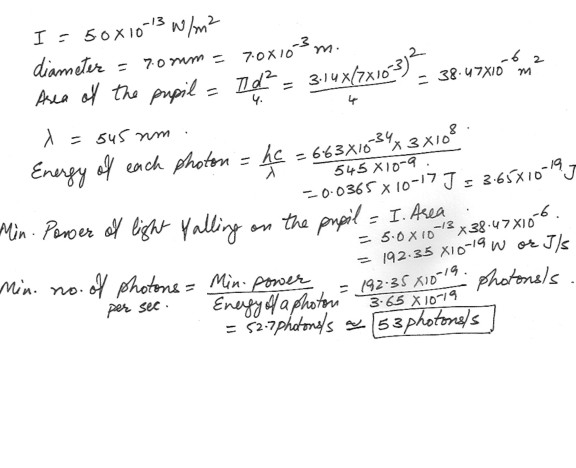2432

Physics Wave Optics Level: High School

An ordinary bright star easily visible to the naked eye emits radiation such that the intensity at the surface of the earth is I = 1.6 x 10^-9 W/m^2 at a wavelength of 560 nm . Estimate the rate at which photons enter the night-adapted eye from such a star . Assume that the pupil diameter is circular , with a diameter of 0.5 cm .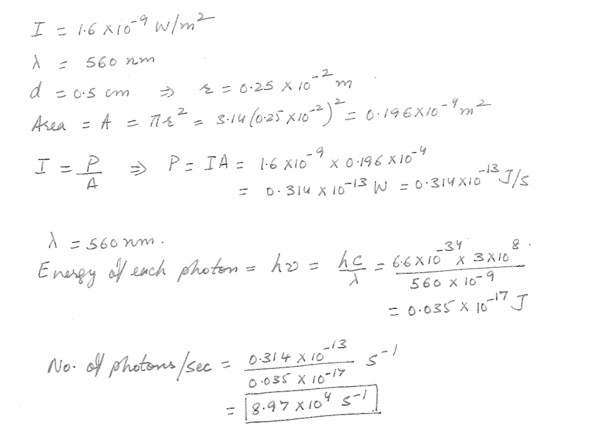Displaying 76-84 of 84 results.